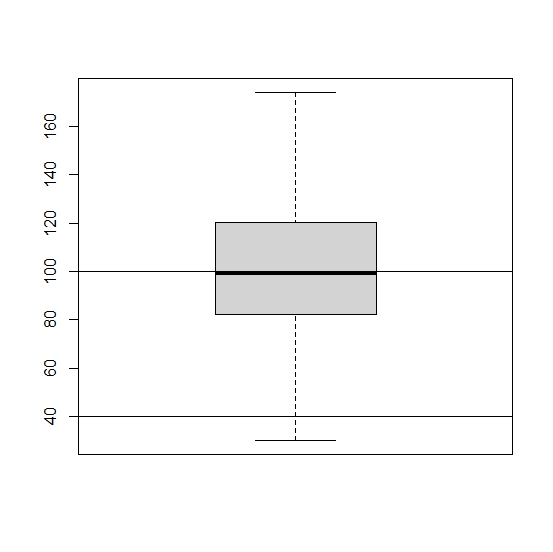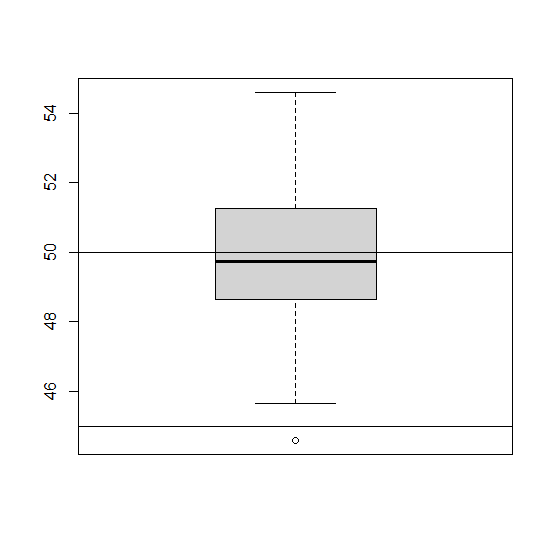# How to create a rectangle inside boxplot in base R?

To create a rectangle inside boxplot in base R, we can use rect function after creating the boxplot. The rect function has many arguments but for the creation of a rectangle only first four are necessary and these are defined as xleft - a vector (or scalar) of left x positions, ybottom - a vector (or scalar) of bottom y positions, xright - a vector (or scalar) of right x positions and ytop - a vector (or scalar) of top y positions.

## Example

Live Demo

x<-rnorm(200,100,25)
boxplot(x)
rect(0,40,50,100)

## Output## Example

Live Demo

y<-rnorm(100,50,2)
boxplot(y)
rect(0,45,50,50)

## Output